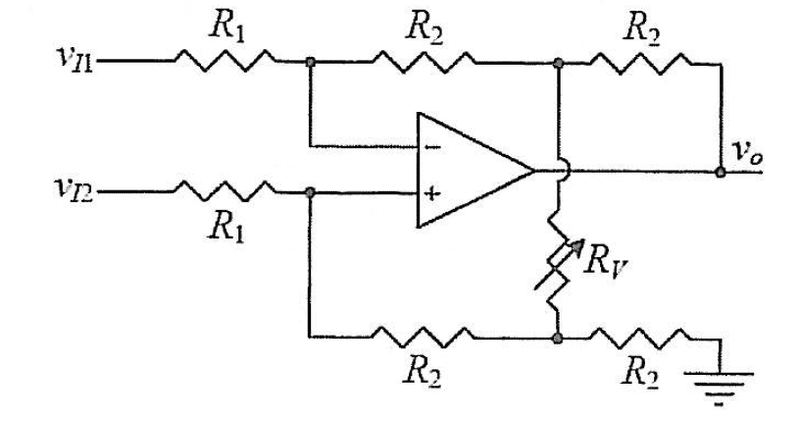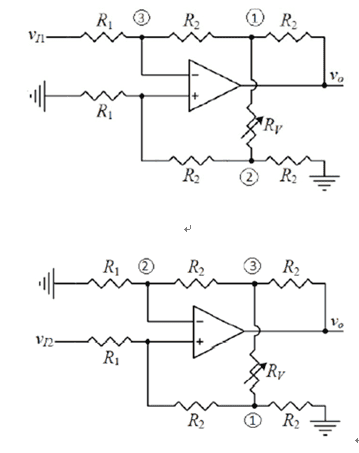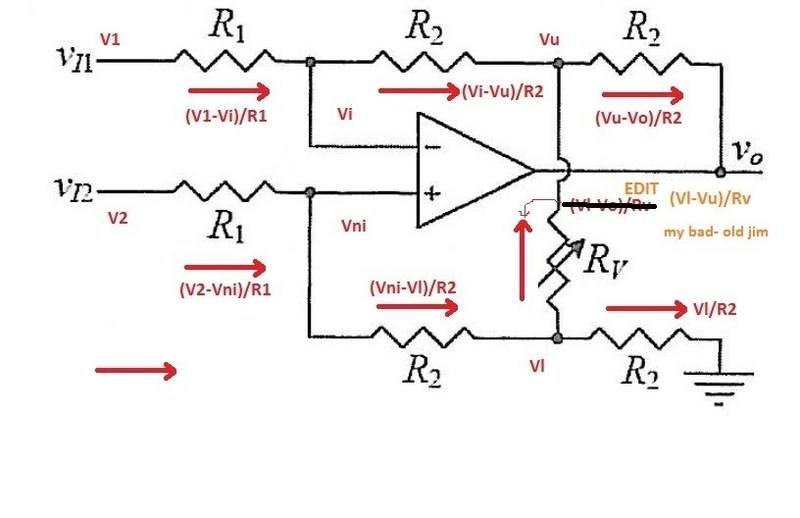# Opamp transfer function problem

• kenok1216

## Homework Statementto prove vo=(2R2/R1)[1+R2/Rv][vI2-vI1] and Rv is variable resistor

## The Attempt at a Solutionupper: 2 is 0V? and then Rv,2R2 form a t-network?
bottom:3 is 0V? if not how can i calculate?[/B]

upper: 2 is 0V? and then Rv,2R2 form a t-network?
No, you don't know what the current from (3) to (2) might be. So you cannot say that V(2) is zero.
bottom:3 is 0V? if not how can i calculate?
No, you cannot know V(3) without knowing both vo and vI2.

You'll need to analyze the circuit from first principles, perhaps using nodal analysis and the properties of an ideal op-amp.

I think, it is a good approach to use superposition - as proposed by you (setting one of both input signals to zero).
Nevertheless, the remaining circuits are still pretty involved and - as suggested by gneill - you should use nodal analyses for both solutions.

If you read this post earlier be aware it had a mistake
which is now corrected, in orange ... old jim

They teach us to solve opamp problems by resolving Zfeedback/Zinput
but when you've got two inputs like above, it's not apparent where to start.

Terminology is important - be picayune and exact with your definitions. I usually quote Lavoisier at this point but will spare you that boring lecture today.

Old JIm says:
An "Operational Amplifier" is just a piece of hardware like a LM741, usually a DC coupled amplifier with high gain
An "Operational Amplifier Circuit " is an electronic circuit , usually having an operational amplifier at its heart,
wired up so that it has a transfer function that's some mathematical operation .like add, multiply, divide, or perform some transcendental function
so we say it "operates" in a mathematical sense not just a "does it work" sense..
That's being nitpicky. But it helps me think straight.

Here are two ground rules i use for op-amp problems.
1. An "operational amplifier circuit " operates er, works by forcing the two inputs to its "operational amplifier" equal
2. It is the duty of the circuit designer to surround the "operational amplifier" with a circuit that let's it do that - if he fails , the circuit will neither "work" nor "operate" .

So let's draw your circuit and label some nodes for convenience
Let's call the voltages at nodes where Rv joins the R2's Vu(pper) and Vl(ower)
and voltages at opamp input nodes Vi(nvertng) and Vn(oninverting)What do we know? What remains unknown ?
V1 and V2 are inputs, so they aren't unknowns
All resistor values are known, if only by their identifying labels

Well,
if the circuit "operates" then Vi = Vni
meaning Vi and Vni are unknown but equal,

so they count as only one unknown Let's call it Vx

Vu and Vl are unknown, so is Vo

So i see four unknowns. Vu, Vl, Vo and Vx.

Can we write four equations to solve for those four unknowns ?

KCL says we can sum currents at nodes Vu and Vl
EDIT , to fix a mistake on my drawing (Thanks Tom G ! )
node Vu: (Vx - Vu)/R2 + (Vi -Vo)/Rv - (Vu - Vo)/R2 = 0
node Vu: (Vx - Vu)/R2 + (Vl-Vu)/Rv - (Vu - Vo)/R2 = 0
node Vl: (Vx - Vl)/R2 - (Vl - Vo)/Rv - Vl/R2 = 0
node Vl: (Vx - Vl)/R2 - (Vl - Vu)/Rv - Vl/R2 = 0

Since input current to the operational amplifier at the heart of this operational amplifier circuit is zero,
Again using KCL , summing currents at nodes Vi and Vni gives
node Vi: (V1 - Vx)/R1 - (Vx - Vu)/R2 = 0
node Vni: (V2 - Vx)/R1 - (Vx - Vl)/R2 = 0

I count four equations and four unknowns... should be an algebra problem from there...
You're looking for Vo as a function of V1, V2 and the three resistor values R1 R2 and Rv.

Now - my algebra is rusty and i get absolutely frustrated trying to do it on a keyboard
so i'd do it longhand several times and see if i get same answer.

That is my "Poor Man's approach" to Opamp circuits:
Set voltage at amplifier inputs equal and solve using basic circuit analysis.

(in my case "Poor Man" meaning one like me who was endowed with a meager portion of mathematical ability)

Let me know if those four equations aren't adequate. Re-Derive them yourself.

Remember that key point - opamp must be allowed to force its inputs equal else the circuit can't "operate" .

i hope this helps you get off the ground and fly through your course. I remember when the light clicked on for me.

old jim

EDIT Tom G found my mistake , which i corrected above
...
thanks Tom

old jim

Last edited:
Post #4 had a mistake in the KCL equations, now fixed..

old jim

They teach us to solve opamp problems by resolving Zfeedback/Zinput
but when you've got two inputs like above, it's not apparent where to start.

Terminology is important - be picayune and

exact with your definitions. I usually quote Lavoisier at this point but will spare you that boring lecture today.

Old JIm says:
An "Operational Amplifier" is just a piece of hardware like a LM741, usually a DC coupled amplifier with high gain
An "Operational Amplifier Circuit " is an electronic circuit , usually having an operational amplifier at its heart,
wired up so that it has a transfer function that's some mathematical operation .like add, multiply, divide, or perform some transcendental function
so we say it "operates" in a mathematical sense not just a "does it work" sense..
That's being nitpicky. But it helps me think straight.

Here are two ground rules i use for op-amp problems.
1. An "operational amplifier circuit " operates er, works by forcing the two inputs to its "operational amplifier" equal
2. It is the duty of the circuit designer to surround the "operational amplifier" with a circuit that let's it do that - if he fails , the circuit will neither "work" nor "operate" .

So let's draw your circuit and label some nodes for convenience
https://www.physicsforums.com/attachments/99799

What do we know? What remains unknown ?
V1 and V2 are inputs, so they aren't unknowns
All resistor values are known, if only by their identifying labels

Well,
if the circuit "operates" then Vi = Vni
meaning Vi and Vni are unknown but equal,

so they count as only one unknown Let's call it Vx
Vu and Vl are unknown, so is Vo

So i see four unknowns. Vu, Vl, Vo and Vx.

Can we write four equations to solve for those four unknowns ?

KCL says we can sum currents at nodes Vu and Vl
node Vu: (Vx - Vu)/R2 + (Vi -Vo)/Rv - (Vu - Vo)/R2 = 0
node Vl: (Vx - Vl)/R2 - (Vl - Vo)/Rv - Vl/R2 = 0

Since input current to the operational amplifier at the heart of this operational amplifier circuit is zero,
Again using KCL , summing currents at nodes Vi and Vni gives
node Vi: (V1 - Vx)/R1 - (Vx - Vu)/R2 = 0
node Vni: (V2 - Vx)/R1 - (Vx - Vl)/R2 = 0

I count four equations and four unknowns... should be an algebra problem from there...
You're looking for Vo as a function of V1, V2 and the three resistor values R1 R2 and Rv.

Now - my algebra is rusty and i get absolutely frustrated trying to do it on a keyboard
so i'd do it longhand several times and see if i get same answer.

That is my "Poor Man's approach" to Opamp circuits:

(in my case "Poor Man" meaning one like me who was endowed with a meager portion of mathematical ability)

Let me know if those four equations aren't adequate. Re-Derive them yourself.

Remember that key point - opamp must be allowed to force its inputs equal else the circuit can't "operate" .

i hope this helps you get off the ground and fly through your course. I remember when the light clicked on for me.

old jim
No, you don't know what the current from (3) to (2) might be. So you cannot say that V(2) is zero.

No, you cannot know V(3) without knowing both vo and vI2.

You'll need to analyze the circuit from first principles, perhaps using nodal analysis and the properties of an ideal op-amp.
I think, it is a good approach to use superposition - as proposed by you (setting one of both input signals to zero).
Nevertheless, the remaining circuits are still pretty involved and - as suggested by gneill - you should use nodal analyses for both solutions.
Post #4 had a mistake in the KCL equations, now fixed..
•